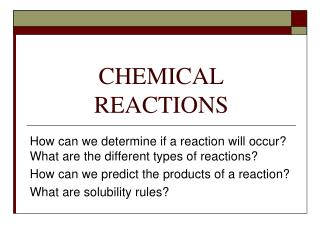DownloadDownload PresentationCHEMICAL REACTIONS

# CHEMICAL REACTIONS

Télécharger la présentation## CHEMICAL REACTIONS

- - - - - - - - - - - - - - - - - - - - - - - - - - - E N D - - - - - - - - - - - - - - - - - - - - - - - - - - -
##### Presentation Transcript

1. CHEMICAL REACTIONS How can we determine if a reaction will occur? What are the different types of reactions? How can we predict the products of a reaction? What are solubility rules?

2. Will a reaction occur? • If a solid is formed (a precipitate) • If water is formed • If a gas is formed • If electrons are transferred Then it is likely that a chemical reaction took place. • Also, if a new odor is produced, a new color is produced, or a change in energy (temperature, light, sound) occurs it is likely that a reaction occurred.

3. Types of Chemical Reactions • Synthesis (composition) • Decomposition • Single Replacement • Double Replacement (precipitation) • Combustion • Redox (reactions involving electron transfer) • Acid-Base Reactions

4. Your Book Classifies as: • Precipitation (Double Replacement) • Oxidation Reduction or Redox (Combustion, Synthesis, Single Replacement, & Decomposition • Acid-Base

5. Predicting products of Double Replacement Reactions • If a solid forms from the combining of two aqueous solutions, a reaction has occurred. • To determine what the solid is, we must know solubility rules. • These are rules that indicate which ionic compounds are soluble in water and which ones are not.

6. Solubility Rules for Ionic Compounds • Compounds containing the following ions are generally soluble in water: • Alkali metal ions and ammonium ions • Acetate ions • Nitrate ions • Halide ions F-, Cl-, Br-, and I- (EXCEPT those containing Ag+, Hg22+,, and Pb2+ )

7. Solubility Continued • Sulfate ions (EXCEPT Ca2+, Sr2+ , Ba2+, Ag+, Hg2+, and Pb2+) • The following are also soluble in water • Strong acids (HCl, HBr, HNO3, H2SO4, HClO3) • Strong bases (LiOH, NaOH, KOH, Ba(OH)2, Ca(OH)2 )

8. Some Insoluble Compounds • Sulfide, carbonate, and phosphate salts, EXCEPT the ones previously mentioned • Hydroxide compounds EXCEPT the ones previously mentioned

9. An Example • Sodium carbonate solution is poured into a calcium nitrate solution. Does a reaction occur? What is the equation if it does?

10. Does it occur? • Look at the two possible products (the rearranged ions). These are sodium nitrate and calcium carbonate. • Are either of these NOT soluble in water? • Calcium carbonate is not soluble in water, so yes this reaction produces a precipitate (turns cloudy and then settles out).

11. The Equation • Na2CO3 + Ca(NO3)2 2NaNO3 + CaCO3

12. You Try This One • Ammonium hydroxide solution is mixed with lead (II) phosphate solution. • Does a reaction occur? • If it does, what is the equation for it?

13. Six More…(4 of these react) • Barium nitrate solution in potassium sulfate solution • Silver nitrate solution in sodium bromide solution • Sodium nitrate solution is iron (II) acetate solution • Iron (III) chloride solution in potassium hydroxide solution • Sodium fluoride solution in sodium sulfate solution • Lead (II) nitrate solution in potassium sulfate solution

14. Predicting Products of Combustion • Always water and carbon dioxide • Is a type of redox

15. Single Replacement ( a type of Redox) • Use a metal activity series to determine if a reaction will occur. • If it will, cations trade places on the product side of the equation. • Ex. Mg + FeCl2 MgCl2 + Fe • Magnesium is more reactive than iron, so it replaces it and forms magnesium chloride

16. Synthesis ( a type of Redox) • Balance the charges of the reactants to form the product • Example: Mg + Cl2 MgCl2

17. Decomposition ( a type of Redox) • One reactant breaks into 2 or more products • Sometimes the component elements, but not always. • Examples: • 2H2O  2H2 + O2 • CuCO3  CuO + CO2